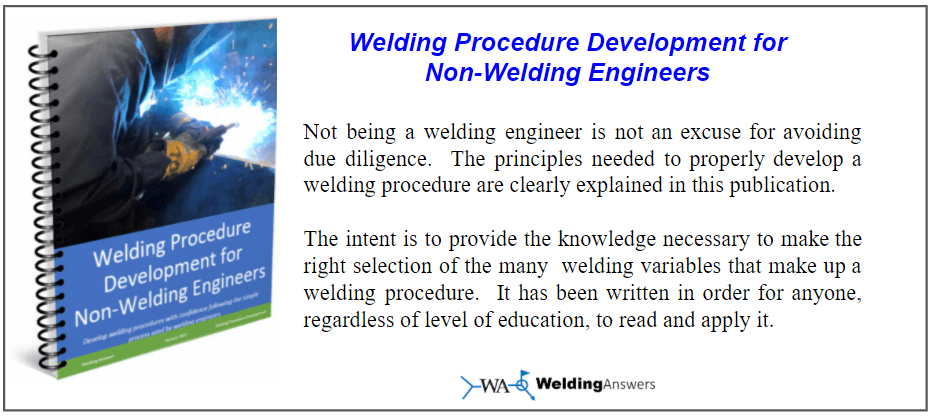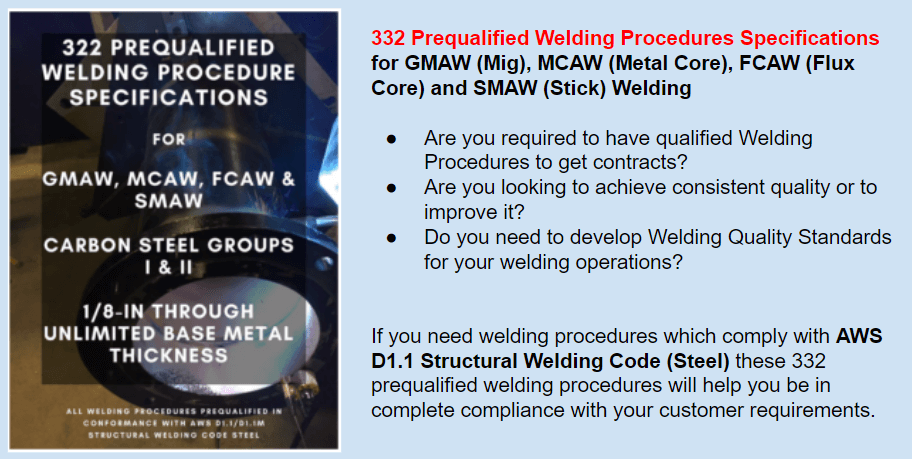# How To Calculate Heat Input From Welding

The vast majority of fabricators are not typically concerned with heat input. For the most part this is OK. But when you are welding on materials whose microstructure can be significantly affected by welding procedures it is important to know about heat input. The reason why heat input is critical in certain applications is because it has a huge bearing on the cooling rate. Typically, faster cooling rates are detrimental to a weldment because they cause embrittlement in the heat affected zone. An example of this is when dealing with materials susceptible to hydrogen-induced cracking in which adequate heat input is critical.

So how do we calculate heat input. It may be a lot easier than you think. If your welding machine has digital or analog meters you are all set. Simply take the reading of amperage and voltage while welding and divide it by the travel speed to get your heat input in joules per inch. The formula is as follows:Heat Input = (60 x Amps x Volts) / (1,000 x Travel Speed in in/min) = KJ/in

The 60 and the 1,000 are there to turn the final answers into Kilojoules per inch.
Example 1: You are welding at 500 inches per minute and 29 volts. Your weld is 25 inches long and it takes the welder 2 minutes to weld it. While he is welding, you notice the machine is displaying an amperage of 325. What is your heat input? Before calculating heat input you need to determine your travel speed.

Travel Speed = Length of Weld / Time to weld = 25 inches / 2 minutes = 12.5 inches per minute

Heat Input = [(60 sec/min) x (325 amps) x (29 volts)] / [(1,000 joules/kilojoule) x (12.5 inches/minute)]
= 45.24 KJ/in

Example 2: Your heat input at 45.24 is too high, but you also notice your weld is oversized. Instead of lowering your amperage and voltage you decide to travel faster. You now complete the same weld in 90 seconds at the exact same parameters. What is your heat input now?
Travel Speed = 25 inches / 1.5 minutes = 16.7 inches/minute

Heat Input = [(60 sec/min) x (325 amps) x (29 volts)] / [(1,000 joules/kilojoule) x (16.7 inches/minute)]
= 33.86 KJ/in

As you can see your heat input dropped by almost 25% simply by traveling faster. Overwelding is a very common problem. Before reducing your amperage and voltage to get lower heat input, see if you can travel faster and deposit a smaller weld.

The use of waveforms in welding is all over the world now.Running pulse programs or even some of the fancier, more advanced, waveforms such as Rapid Arc® from Lincoln Electric or Accu Pulse® from Miller Electric, is common practice. So how do you calculate heat input when you are pulsing and your amperage is all over the place (fluctuating between a peak and a background current)?Newer welding machines have the ability of displaying total energy of each and every weld that you make.

Some welding machines will give you an actual value for either total energy for a weld, or instantaneous energy (energy at any given time). You then would have to do some quick math to get your heat input.

Example 3: You are using a special waveform on a Lincoln Electric Power Wave S500 power source. The readout on the display says that the total energy for your 25 inch weld was 459 KJ. What is your heat input in KJ/in? In this case you don’t need to worry about your travel speed (at least for the heat input calculation).

Heat Input = Total energy / length of weld = 459KJ / 25 inches = 18.36 KJ/in.

So what do you do if you are pulse welding but your welder does not give you total energy? Some welders will display average amperage as you are welding. In this case simply take that amperage and follow the formula on example 1 and 2. If the meter varies between peak  and background amperage you will have to look at the highest and lowest reading and take an average. Keep in mind that this may not yield an accurate result, but it will get you close.

If your machine does not have meters you may need to use Fluke Meter or other voltage/amperage meter to take readings or simply use a different machine.

References:
Effect of Heat Input on Residual Stress in Submerged Arc Welds – R.K. Saxena
The Procedure Handbook of Arc Welding, 14th Edition
New Code Requirements for Calculating Heat Input- The Welding Journal, June 201o – Theresa MelfiAre you in charge of performing welding procedures and welder qualifications?  Are you working with AWS D1.1 Structural Welding Code – Steel?  Are you taking advantage of prequalified welding procedures?

“Qualifying Welding Procedures, Welders and Welding Operators” is a guide developed to help you perform your own qualifications in full compliance to AWS D1.1 Structural Welding Code.

Please note: I reserve the right to delete comments that are offensive or off-topic.

## 16 thoughts on “How To Calculate Heat Input From Welding”

1.Agustin Abaroa says:

Do you have procedures for cast iron welding?

2.zahid says:

Sir, My question is about Arc Energy and Total Energy, Are these two terms are the same or they have different meaning. Since Arc Energy multiplied with Thermal Efficiency gives Heat Input. where as Total Energy Divided by Length of weld also gives Heat Input.

•Welding Answers says:

Total energy is the amount of energy (joules) for a weld. Arc energy can vary with changes in amperage, it is basically amps x volts at any given time.

3.Petrus says:

i want an answer to my question, if you are running WQT test and welder is using SMAW welding process. he is using electrode of size 2.5mm for rooting he spent 6.8mins for 4 electrodes and test piece length is 300mm. how do you calculate travel speed?

•Welding Answers says:

Hello Petrus. Travel speed is simply distance divided by time. So if it took you 6.8 minutes to travel 300mm your travel speed would be 300mm/6.8min = 44.1 mm/minute. Or 0.735mm/sec. Then simply follow the formula given
HEAT INPUT = (60 x Amps x Volts) / (Travel Speed) = Joules/mm

4.Faraz Ahmed says:

Hello

I am doing a scientific research project on ultrasonic welding of 3d printed ABS parts.
Before welding i have to set the enrgy required for the weld.
How do i determine how much energy i should set in the machine before going through the welding process.
I will be using Hermann ultraschall Dialog welding machine .
Is there any reference for such calculation .

5.Zohaib fazal says:

can you give me this information in pdf file please.?
if yes then this is my Email address
zohaib0525@gmail.com

•Welding Answers says:

Sorry, we do not have these posts available as PDF.

6.Farhan akhtar says:

7.Bongani says:

Good day,
Please assist with method to calculate weld heat input for automotive welding : spot, projection ( Resistance) and arc welding

•The Welding Guys says: